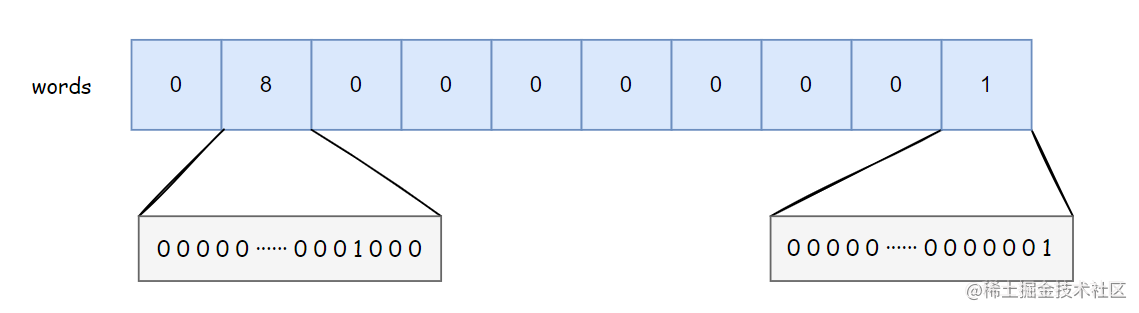# 深入解析 Bitmap 算法（二）：BitSet# 前言

`java.util.BitSet` 是 JDK 中对 `Bitmap` 算法的实现类，使用了 `long[]` 来存储二进制数据。`BitSet` 提供了 添加、删除、获取数据 以及 与、或、异或 等操作。

# 存储结构

`BitSet` 使用一个 `long[]` 来存储数据，`long` 类型占 8 字节，64位。数组中每个元素可以存储 `64` 个数据，数组中数据的存储顺序 从左到右，从低位到高位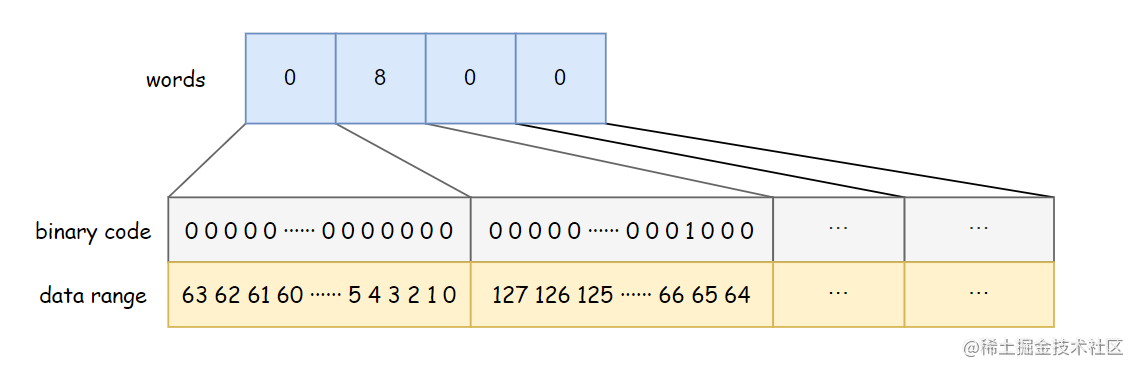`BitSet` 中定义的一些属性如下：

• `long[] words`：数据的存储结构
• `int wordsInUse = 0`：表示数组中最多使用的元素个数，也就是最后一个不为 0 的元素的索引加 1；比如上图中，数组长度为 4，但是最后一个不为 0 的元素是 1，所以 `wordsInUse = 2`

``````// 每个 word 用 6 个地址位来表示，2^6 = 64
private static final int ADDRESS_BITS_PER_WORD = 6;
// 每个 word 所占的位数，即 64
private static final int BITS_PER_WORD = 1 << ADDRESS_BITS_PER_WORD;
// ?
private static final int BIT_INDEX_MASK = BITS_PER_WORD - 1;
// ?
private static final long WORD_MASK = 0xffffffffffffffffL;

# 初始化

``````/**
* BitSet.class
* 创建一个能存储给定数据索引的 BitSet
*/
public BitSet(int nbits) {
// 参数合法性判断
if (nbits < 0)
throw new NegativeArraySizeException("nbits < 0: " + nbits);
// 调用 initWords 方法初始化
initWords(nbits);
sizeIsSticky = true;
}

private void initWords(int nbits) {
words = new long[wordIndex(nbits-1) + 1];
}

`initWords()` 方法非常简单，就是 `new` 一个数组。其中调用了 `wordIndex()` 方法，该方法的作用是 得到所给 `bit` 索引（数据）对应在 words 中的下标

``````// 得到 bitIndex 对应的 words 下标
private static int wordIndex(int bitIndex) {
}

# 添加数据

`set(bitIndex)` 的源码如下：

``````// BitSet.class
public void set(int bitIndex) {
// 参数合法性检验
if (bitIndex < 0)
throw new IndexOutOfBoundsException("bitIndex < 0: " + bitIndex);
// 得到对应的数组下标
int wordIndex = wordIndex(bitIndex);
// 是否要扩容
expandTo(wordIndex);
// 修改数据
words[wordIndex] |= (1L << bitIndex);
// 参数检查
checkInvariants();
}

``````private void expandTo(int wordIndex) {
int wordsRequired = wordIndex+1;
if (wordsInUse < wordsRequired) {
ensureCapacity(wordsRequired);
wordsInUse = wordsRequired;
}
}

``````1L << bitIndex <=> 1L << bitIndex % 64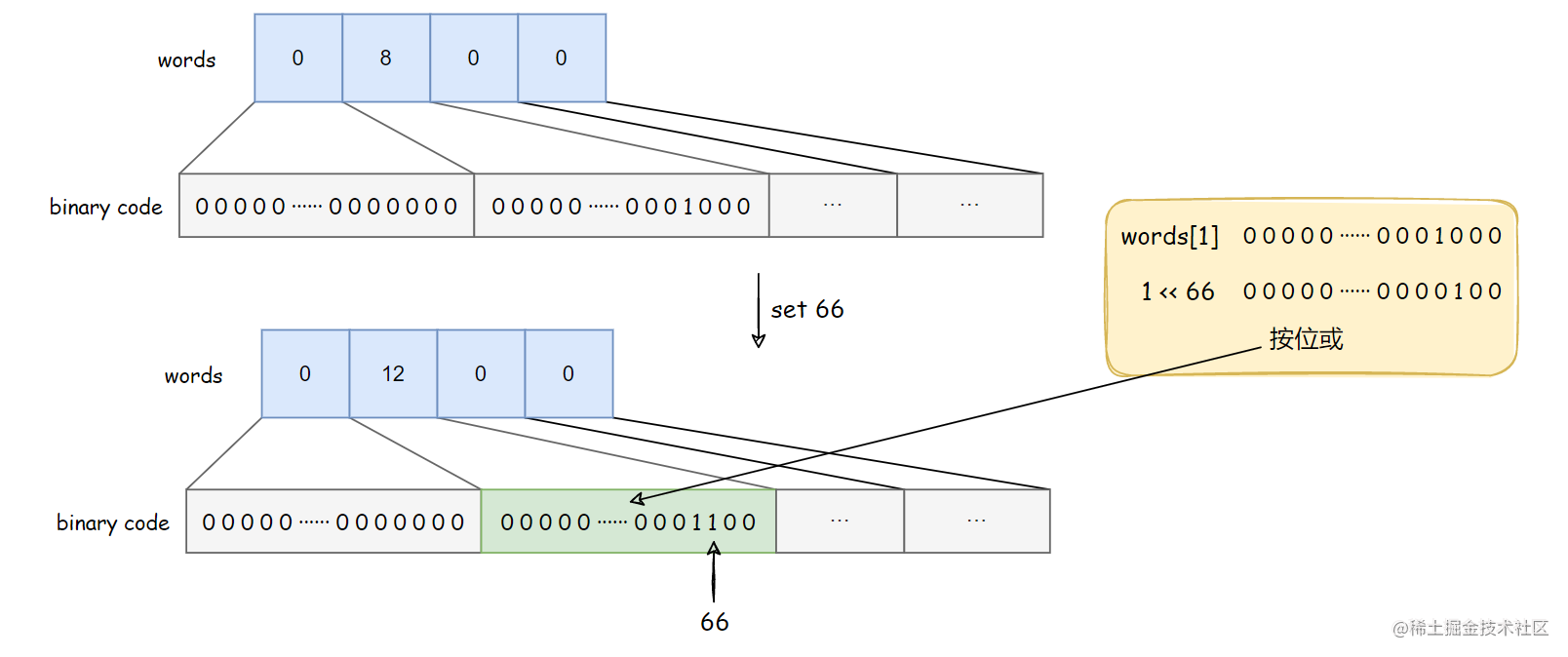# 清除数据

``````/**
* BitSet.class
* clear 核心代码
*/
public void clear(int bitIndex) {
//...
int wordIndex = wordIndex(bitIndex);
// 如果 wordIndex >= wordsInUse，说明该索引要么不存在，要么一定是 0 ，直接返回即可
if (wordIndex >= wordsInUse)
return;
words[wordIndex] &= ~(1L << bitIndex);
recalculateWordsInUse();
//...
}

``````words[wordIndex] &= ~(1L << bitIndex);

``````private void recalculateWordsInUse() {
int i;
for (i = wordsInUse-1; i >= 0; i--)
if (words[i] != 0)
break;

wordsInUse = i+1; // The new logical size
}

# 获取数据

``````/**
* BitSet.class
* get() 核心代码
*/
public boolean get(int bitIndex) {
// ...
int wordIndex = wordIndex(bitIndex);
return (wordIndex < wordsInUse) && ((words[wordIndex] & (1L << bitIndex)) != 0);
}

`get(int)` 方法判断给定的数据索引 `bitIndex` 是否存在，也就是对应二进制位是否为 `1`

``````words[wordIndex] & (1L << bitIndex)) != 0

# 集合操作

## 与操作

``````public void and(BitSet set) {
if (this == set)
return;
while (wordsInUse > set.wordsInUse)
words[--wordsInUse] = 0;
for (int i = 0; i < wordsInUse; i++)
words[i] &= set.words[i];

recalculateWordsInUse();
checkInvariants();
}

## 或操作

``````/**
* BitSet.class
*/
public void or(BitSet set) {
if (this == set)
return;
// 获取公共部分范围
int wordsInCommon = Math.min(wordsInUse, set.wordsInUse);
// 必要时扩容
if (wordsInUse < set.wordsInUse) {
ensureCapacity(set.wordsInUse);
wordsInUse = set.wordsInUse;
}
// 公共部分按位或
for (int i = 0; i < wordsInCommon; i++)
words[i] |= set.words[i];
// 复制剩余元素
if (wordsInCommon < set.wordsInUse)
System.arraycopy(set.words, wordsInCommon,words, wordsInCommon,wordsInUse - wordsInCommon);
}

• 如果 `wordsInUse >= set.wordsInUse` ，这种情况比较简单，不需要做其他额外的操作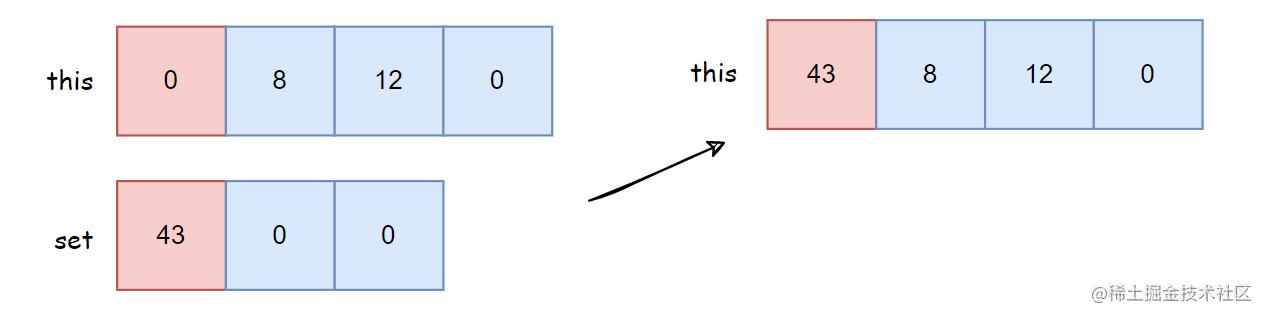• 如果 `wordsInUse < set.wordsInUse`，这种情况下，首先要判断是否需要扩容，然后公共部分（下图红色部分）按位或，最后将 `set` 中剩余的元素（下图绿色部分）直接复制到 `this` 中。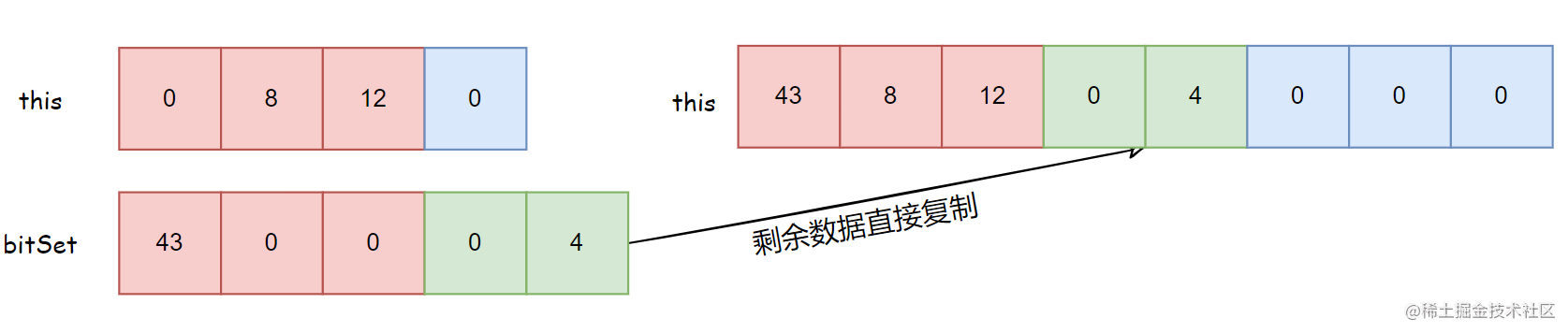## 异或操作

``````/**
* BitSet.class
*/
public void xor(BitSet set) {
// 获取公共部分范围
int wordsInCommon = Math.min(wordsInUse, set.wordsInUse);
// 必要时扩容
if (wordsInUse < set.wordsInUse) {
ensureCapacity(set.wordsInUse);
wordsInUse = set.wordsInUse;
}
// 公共部分异或操作
for (int i = 0; i < wordsInCommon; i++)
words[i] ^= set.words[i];
// 复制剩余元素
if (wordsInCommon < set.wordsInUse)
System.arraycopy(set.words, wordsInCommon,
words, wordsInCommon,
set.wordsInUse - wordsInCommon);
// 重新计算 wordsInUse
recalculateWordsInUse();
checkInvariants();
}

# 总结

`BitSet` 使用 `long` 数组来存储数据，并且使用了一个 `wordsInUse` 变量，能够忽略数组尾部的 0 元素。